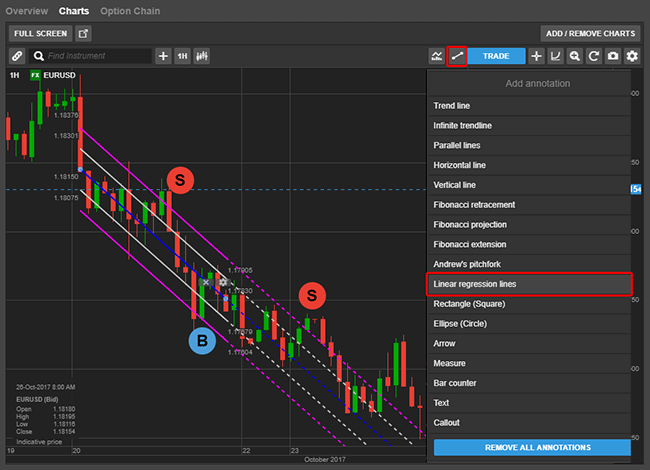# New chart annotation tool - Linear Regression Lines

From 10th November 2017, Linear Regression Lines will be available in SaxoTraderGO. Linear Regression Lines is a new statistical chart annotation tool which tries to predict future values from past values and is a straight line that best fits the prices between a starting price point and an ending price point.

A "best fit" means that a line is constructed where there is the least amount of space between the price points and the actual Linear Regression Line.

Traders use the Linear Regression Lines to try to determine a trend direction regarded as the fair value. If the price for an instrument is below a Linear Regression Line for some time, it could be an indication of a trend change and this could be considered a buy signal. Similarly, if the price is above the Linear Regression Line it could be considered a sell signal.

It can be difficult to identify how far below or above the line a price should move from the Linear Regression Line before it is a good buy or sell signal. For more objective indicators, extra lines can be added parallel to the Linear Regression Channel - we have added extra lines by default at ±1 and ±2 Standard Deviations. You can remove these outer lines in the configuration settings for the annotation.

The following EURUSD chart shows a Linear Regression Channel with the lines extended (shown as dashed lines).We have indicated a few areas on the chart where it might be a good signal to buy or sell i.e. when the price crosses the outer 2 Standard Deviations line from the Linear Regression.

In the configuration settings for the Linear Regression Line, you can delete or add lines, extend them as well as the usual configuration such as colors, line type and calculation details.